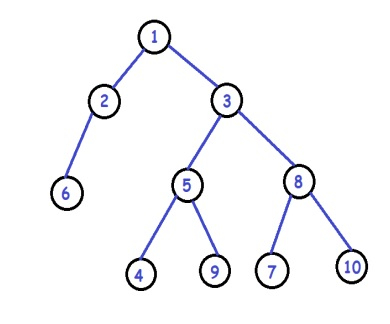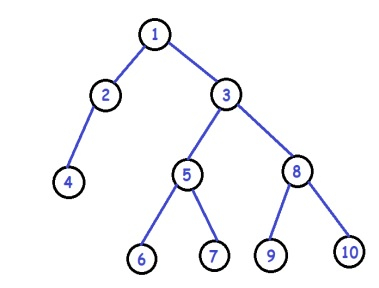# C++ Pairwise Swap Leaf Nodes in a Binary Tree

Given a binary tree. The task is to pairwise swap the leaf nodes, for example −

Input −Output −We will keep track of two pointers that point to the two adjacent leaf nodes and swap their values in the given problem.

## Approach to Find the Solution

In this approach, we traverse the tree, find the leaf nodes, and keep track of our counter to check the current count. The main trick is that our counter is odd, so our first pointer points to that node now. When our counter becomes even, we swap the data, and hence our leaf nodes are swapped.

## Example

C++ Code for the Above Approach

#include <bits/stdc++.h>
using namespace std;
struct Node{ // structure of our tree node
int data;
struct Node *left, *right;
};
void Swap(Node **a, Node **b){ // the swapping utility function
Node * temp = *a;
*a = *b;
*b = temp;
}
/********Pointers for leaf nodes for swapping********/
Node **firstleaf;
Node **secondleaf;
void SwapTheLeafNodes(Node **root, int &count){//recursive function for
//Swapping leaf nodes
if (!(*root)) // if root is null we return
return;
if(!(*root)->left &&!(*root)->right){ // condition for leaf node
secondleaf = root; // now we firstly make our second pointer point to this node
count++; // we also increment the count
if (count%2 == 0) // now if our count is even that means we have a pair so we can swap them
Swap(firstleaf, secondleaf);
else // if count is odd so that means we only got first node yet
firstleaf = secondleaf;
}
if ((*root)->left)
SwapTheLeafNodes(&(*root)->left, count);
if ((*root)->right)
SwapTheLeafNodes(&(*root)->right, count);
}
Node* newNode(int data){ // function for initializing new node
Node *temp = new Node;
temp->data = data;
temp->left = temp->right = NULL;
return temp;
}
void printInorder(Node* node){ // inorder traversal function
if (node == NULL)
return;
printInorder(node->left);
printf("%d ", node->data);
printInorder(node->right);
}
int main(){
/* Creating binary tree*/
Node *root = newNode(1);
root->left = newNode(2);
root->right = newNode(3);
root->left->left = newNode(4);
root->right->left = newNode(5);
root->right->right = newNode(8);
root->right->left->left = newNode(6);
root->right->left->right = newNode(7);
root->right->right->left = newNode(9);
root->right->right->right = newNode(10);
cout << "Inorder traversal before swap:\n";
printInorder(root);
cout << "\n";
int count = 0; // out counter for keeping track of leaf nodes
SwapTheLeafNodes(&root, count); // swapping the nodes
cout << "Inorder traversal after swap:\n";
printInorder(root);
cout << "\n";
return 0;
}

## Output

Inorder traversal before swap:
4 2 1 6 5 7 3 9 8 10
Inorder traversal after swap:
6 2 1 4 5 9 3 7 8 10

## Explanation of the Above Code

In the above approach, we are simply making up two pointers that will keep track of our leaf nodes. We traverse the tree when we encounter a leaf node. We firstly make our second pointer point to that node now we increment a count variable now if our count is even, so we swap the nodes and if the count is odd, so that means we only found the first element of our pair, so we store that value in the first pointer, and this is how our function works.

## Conclusion

In this tutorial, we solve the problem of Pairwise Swap leaf nodes in a binary tree. We also learned the C++ program for this problem and the complete approach ( Normal and efficient ) by which we solved this problem. We can write the same program in other languages such as C, java, python, and other languages. We hope you find this tutorial helpful.